Скачать презентацию Cryptography and Network Security Chapter 13 Fifth Edition

54bd068361780cca006d466465cb700a.ppt

• Количество слайдов: 22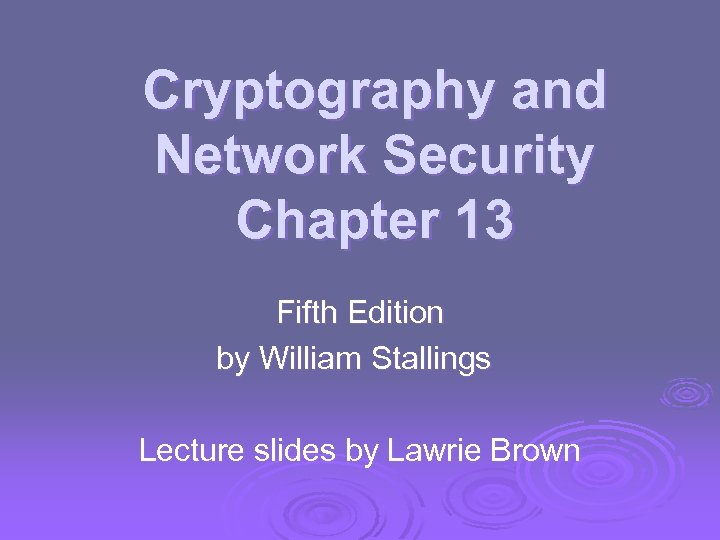Cryptography and Network Security Chapter 13 Fifth Edition by William Stallings Lecture slides by Lawrie Brown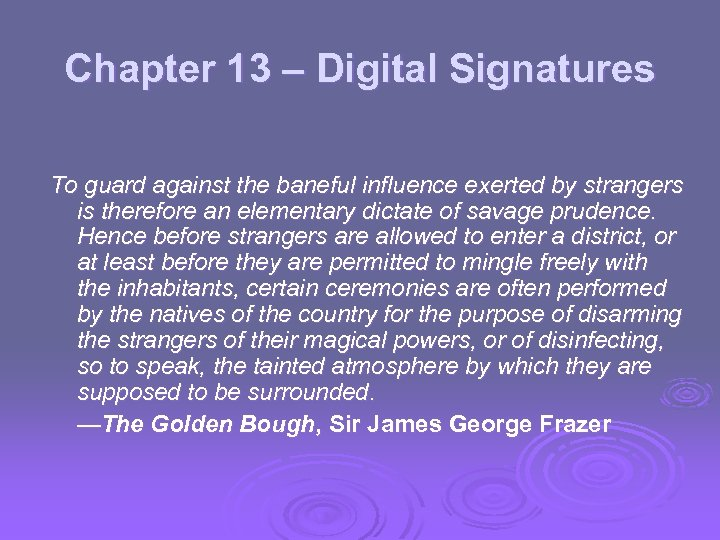Chapter 13 – Digital Signatures To guard against the baneful influence exerted by strangers is therefore an elementary dictate of savage prudence. Hence before strangers are allowed to enter a district, or at least before they are permitted to mingle freely with the inhabitants, certain ceremonies are often performed by the natives of the country for the purpose of disarming the strangers of their magical powers, or of disinfecting, so to speak, the tainted atmosphere by which they are supposed to be surrounded. —The Golden Bough, Sir James George Frazer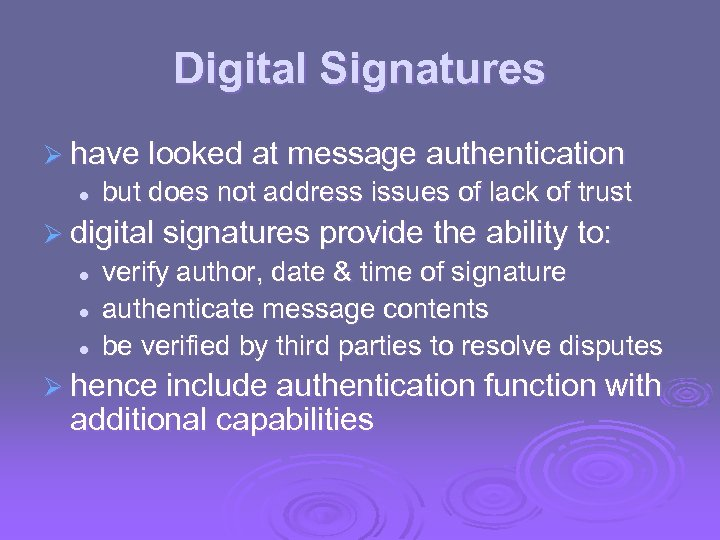Digital Signatures Ø have looked at message authentication l but does not address issues of lack of trust Ø digital signatures provide the ability to: l l l verify author, date & time of signature authenticate message contents be verified by third parties to resolve disputes Ø hence include authentication function with additional capabilities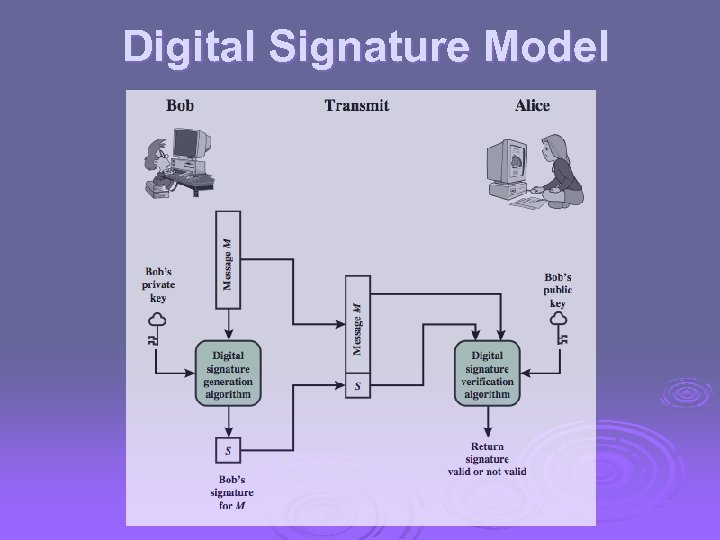Digital Signature Model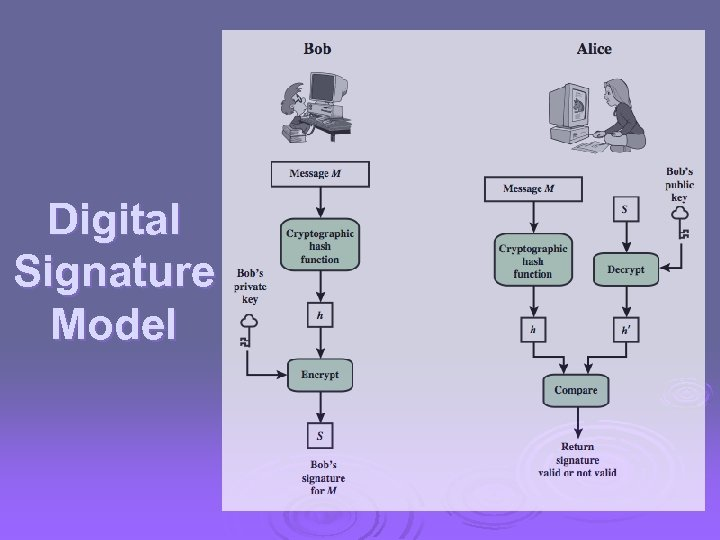Digital Signature Model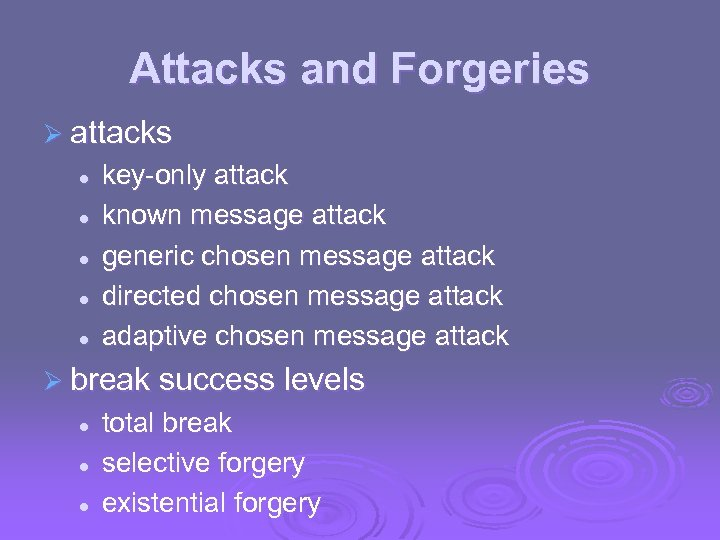Attacks and Forgeries Ø attacks l l l key-only attack known message attack generic chosen message attack directed chosen message attack adaptive chosen message attack Ø break success levels l l l total break selective forgery existential forgery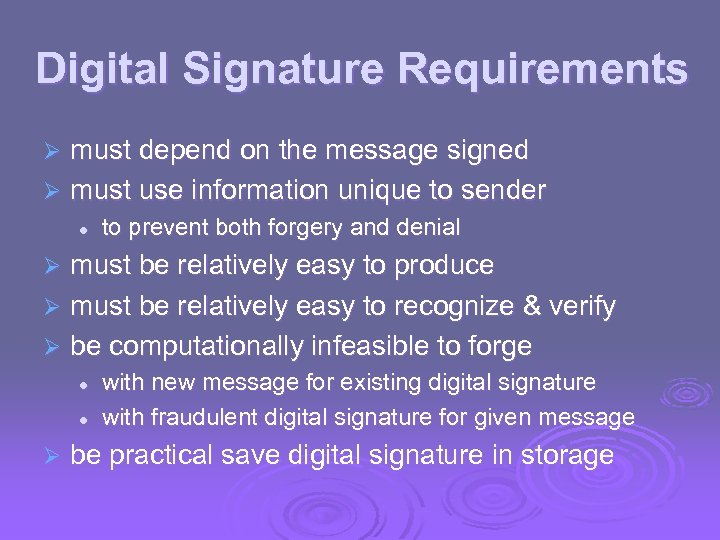Digital Signature Requirements must depend on the message signed Ø must use information unique to sender Ø l to prevent both forgery and denial must be relatively easy to produce Ø must be relatively easy to recognize & verify Ø be computationally infeasible to forge Ø l l Ø with new message for existing digital signature with fraudulent digital signature for given message be practical save digital signature in storage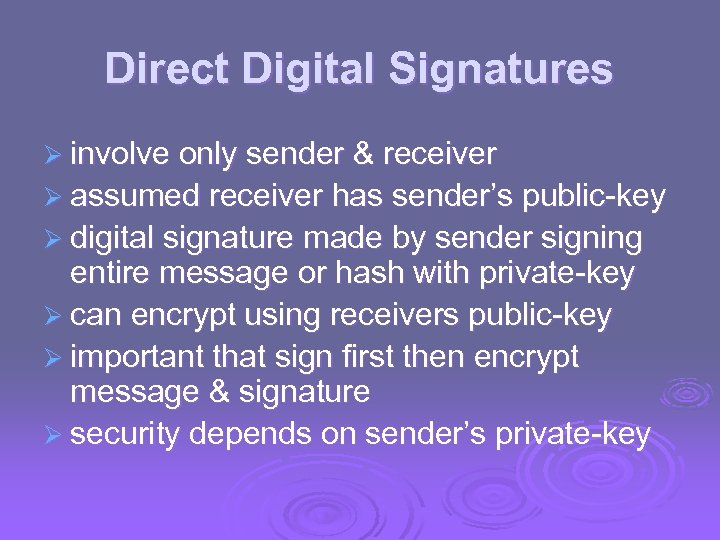Direct Digital Signatures Ø involve only sender & receiver Ø assumed receiver has sender’s public-key Ø digital signature made by sender signing entire message or hash with private-key Ø can encrypt using receivers public-key Ø important that sign first then encrypt message & signature Ø security depends on sender’s private-key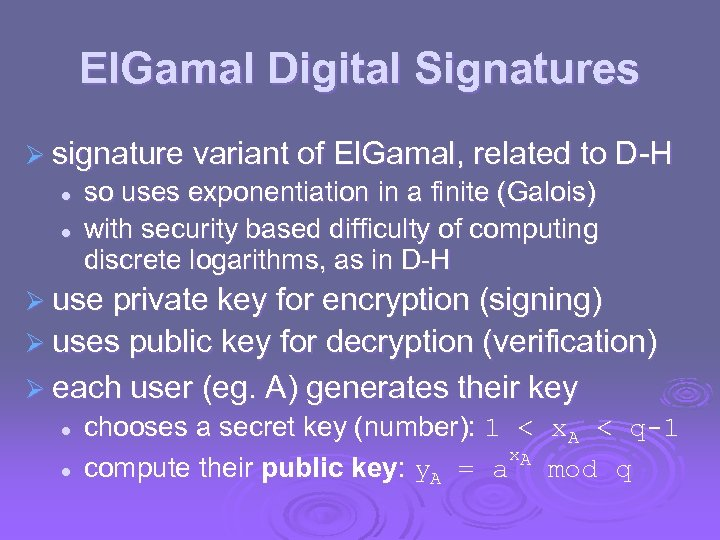El. Gamal Digital Signatures Ø signature variant of El. Gamal, related to D-H l l so uses exponentiation in a finite (Galois) with security based difficulty of computing discrete logarithms, as in D-H Ø use private key for encryption (signing) Ø uses public key for decryption (verification) Ø each user (eg. A) generates their key l l chooses a secret key (number): 1 < x. A < q-1 x. A compute their public key: y. A = a mod q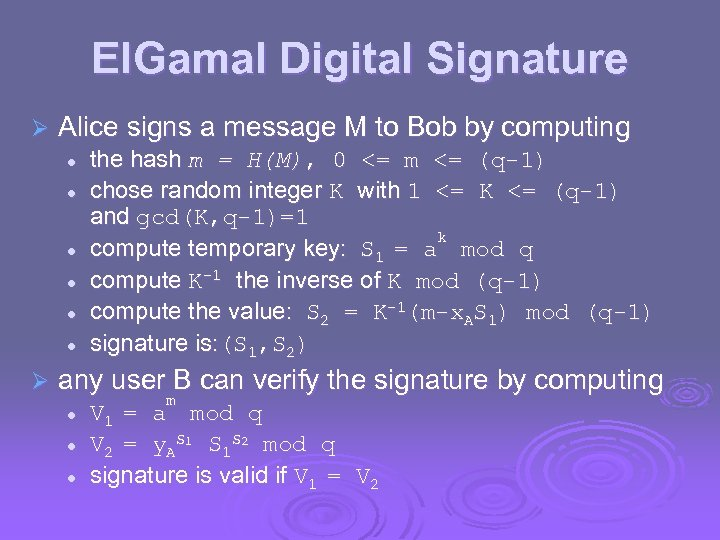El. Gamal Digital Signature Ø Alice signs a message M to Bob by computing l l l Ø the hash m = H(M), 0 <= m <= (q-1) chose random integer K with 1 <= K <= (q-1) and gcd(K, q-1)=1 k compute temporary key: S 1 = a mod q compute K-1 the inverse of K mod (q-1) compute the value: S 2 = K-1(m-x. AS 1) mod (q-1) signature is: (S 1, S 2) any user B can verify the signature by computing l l l m V 1 = a mod q V 2 = y. AS 1 S 1 S 2 mod q signature is valid if V 1 = V 2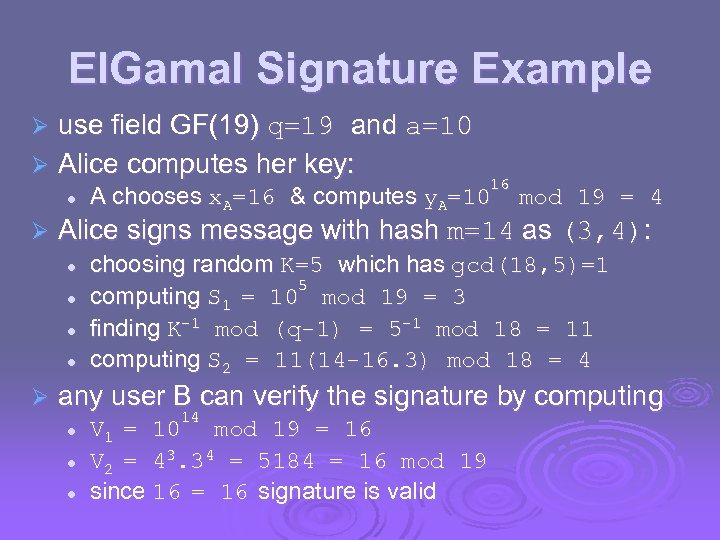El. Gamal Signature Example use field GF(19) q=19 and a=10 Ø Alice computes her key: Ø l Ø mod 19 = 4 Alice signs message with hash m=14 as (3, 4): l l Ø 16 A chooses x. A=16 & computes y. A=10 choosing random K=5 which has gcd(18, 5)=1 5 computing S 1 = 10 mod 19 = 3 finding K-1 mod (q-1) = 5 -1 mod 18 = 11 computing S 2 = 11(14 -16. 3) mod 18 = 4 any user B can verify the signature by computing l l l 14 V 1 = 10 mod 19 = 16 V 2 = 43. 34 = 5184 = 16 mod 19 since 16 = 16 signature is valid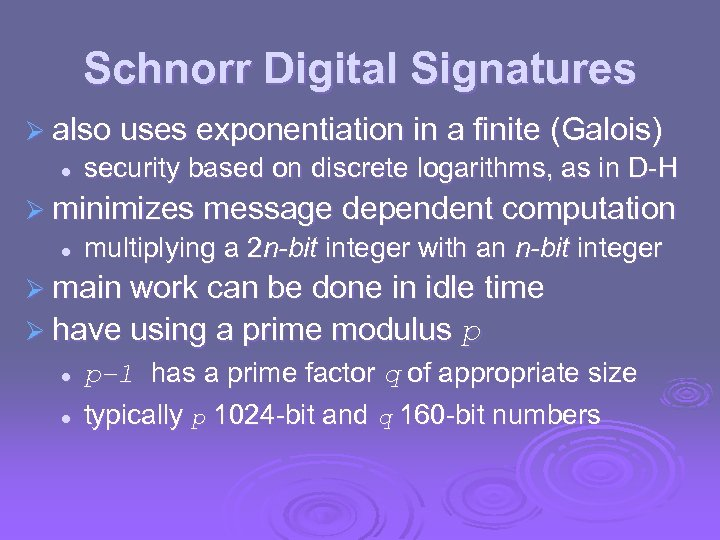Schnorr Digital Signatures Ø also uses exponentiation in a finite (Galois) l security based on discrete logarithms, as in D-H Ø minimizes message dependent computation l multiplying a 2 n-bit integer with an n-bit integer Ø main work can be done in idle time Ø have using a prime modulus p l l p– 1 has a prime factor q of appropriate size typically p 1024 -bit and q 160 -bit numbers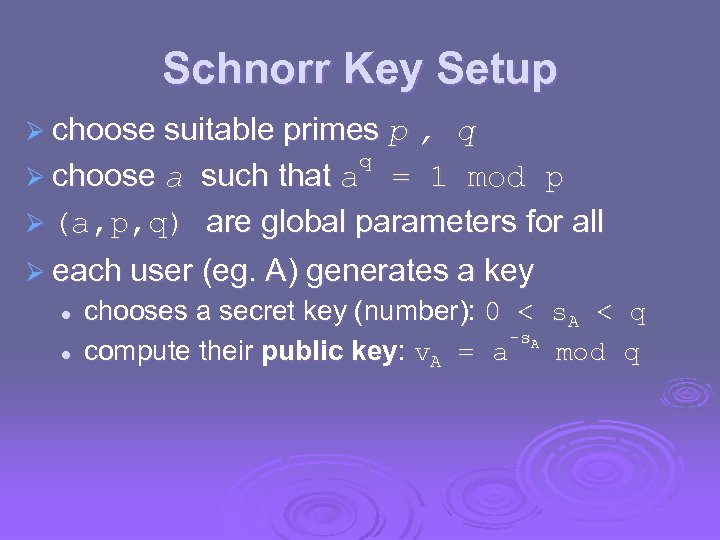Schnorr Key Setup Ø choose suitable primes p , q q Ø choose a such that a = 1 mod p Ø (a, p, q) are global parameters for all Ø each user (eg. A) generates a key l l chooses a secret key (number): 0 < -s. A compute their public key: v. A = a s. A < mod q q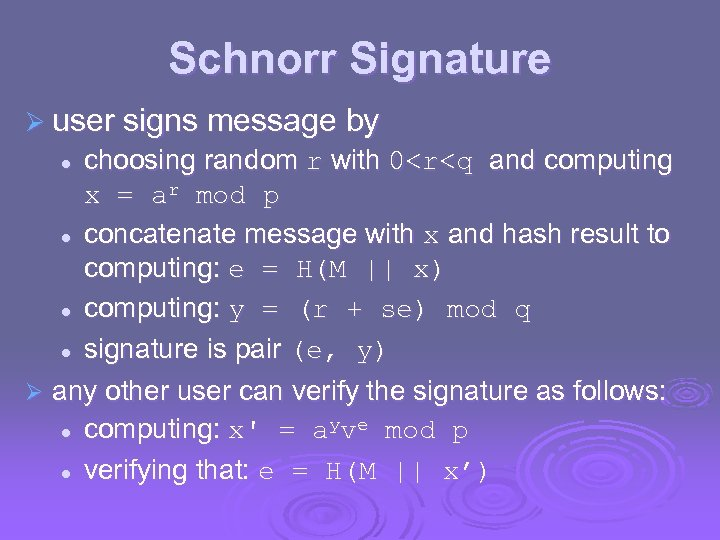Schnorr Signature Ø user signs message by choosing random r with 0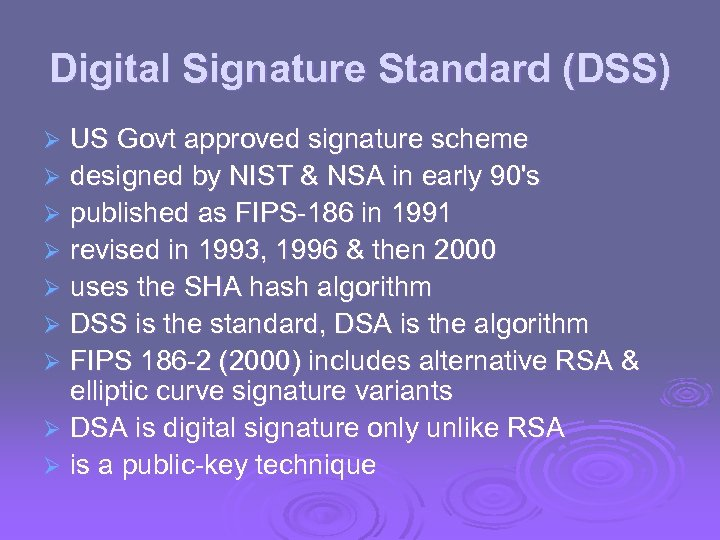Digital Signature Standard (DSS) US Govt approved signature scheme Ø designed by NIST & NSA in early 90's Ø published as FIPS-186 in 1991 Ø revised in 1993, 1996 & then 2000 Ø uses the SHA hash algorithm Ø DSS is the standard, DSA is the algorithm Ø FIPS 186 -2 (2000) includes alternative RSA & elliptic curve signature variants Ø DSA is digital signature only unlike RSA Ø is a public-key technique Ø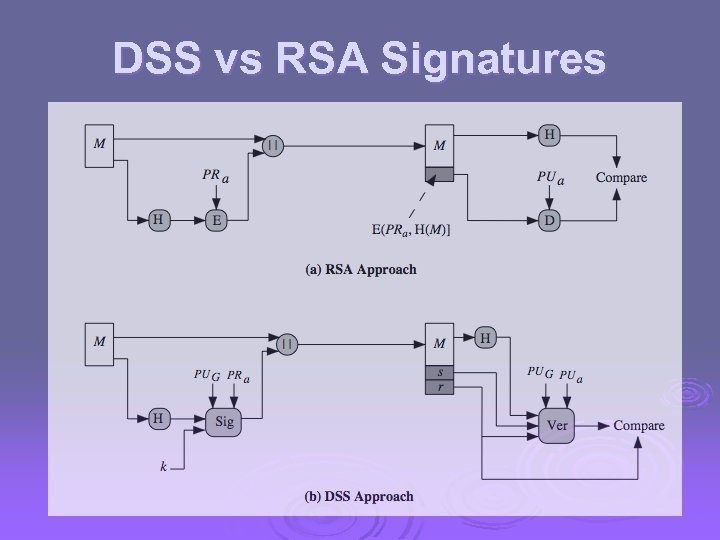DSS vs RSA Signatures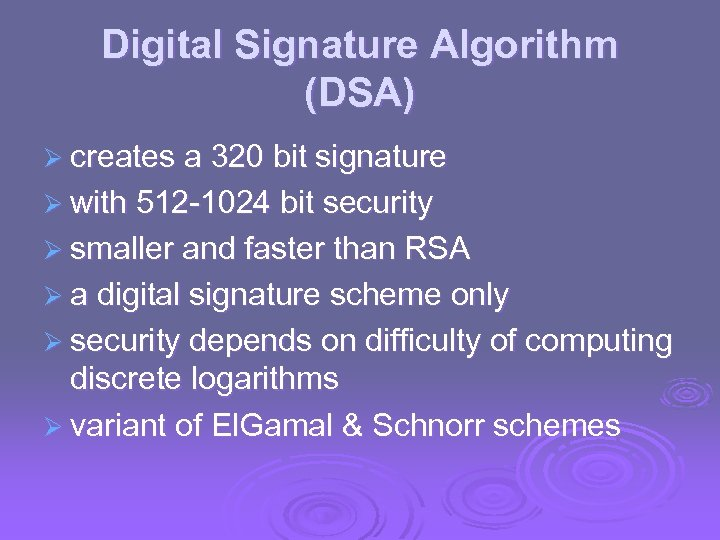Digital Signature Algorithm (DSA) Ø creates a 320 bit signature Ø with 512 -1024 bit security Ø smaller and faster than RSA Ø a digital signature scheme only Ø security depends on difficulty of computing discrete logarithms Ø variant of El. Gamal & Schnorr schemes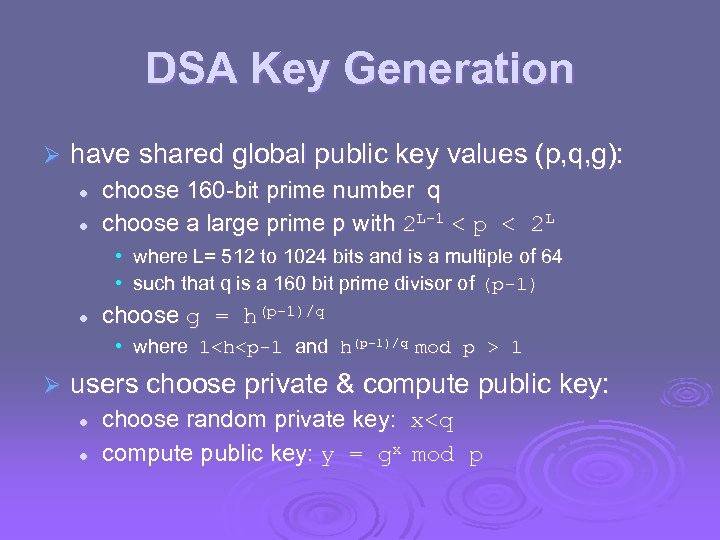DSA Key Generation Ø have shared global public key values (p, q, g): l l choose 160 -bit prime number q choose a large prime p with 2 L-1 < p < 2 L • where L= 512 to 1024 bits and is a multiple of 64 • such that q is a 160 bit prime divisor of (p-1) l choose g = h(p-1)/q • where 1 1 Ø users choose private & compute public key: l l choose random private key: x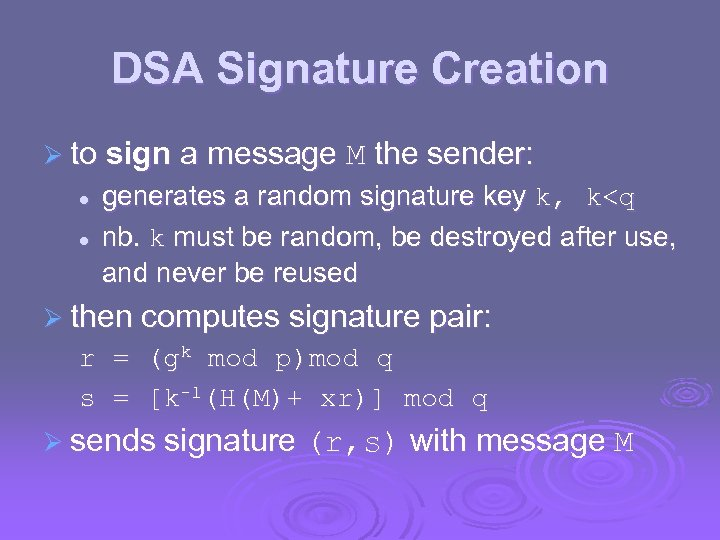DSA Signature Creation Ø to sign a message M the sender: l l generates a random signature key k, k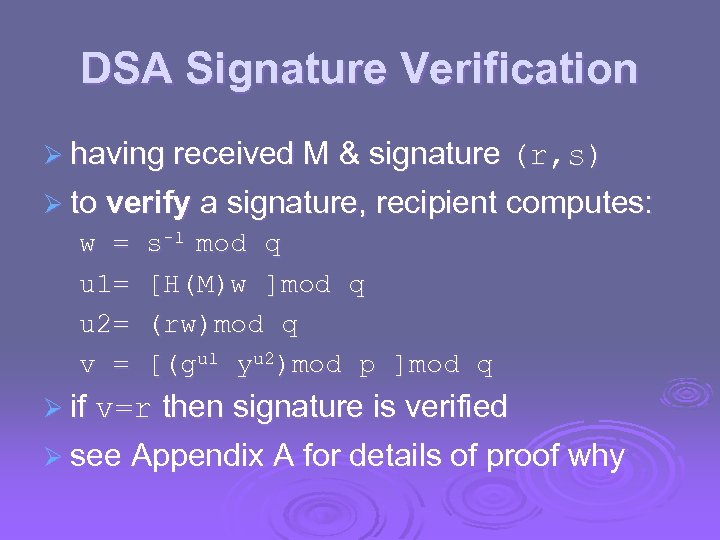DSA Signature Verification Ø having received M & signature (r, s) Ø to verify a signature, recipient computes: w = u 1= u 2= v = s-1 mod q [H(M)w ]mod q (rw)mod q [(gu 1 yu 2)mod p ]mod q Ø if v=r then signature is verified Ø see Appendix A for details of proof why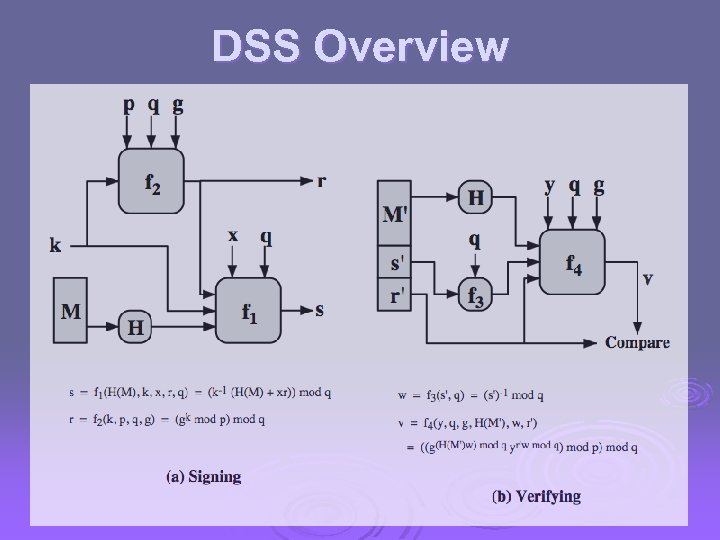DSS Overview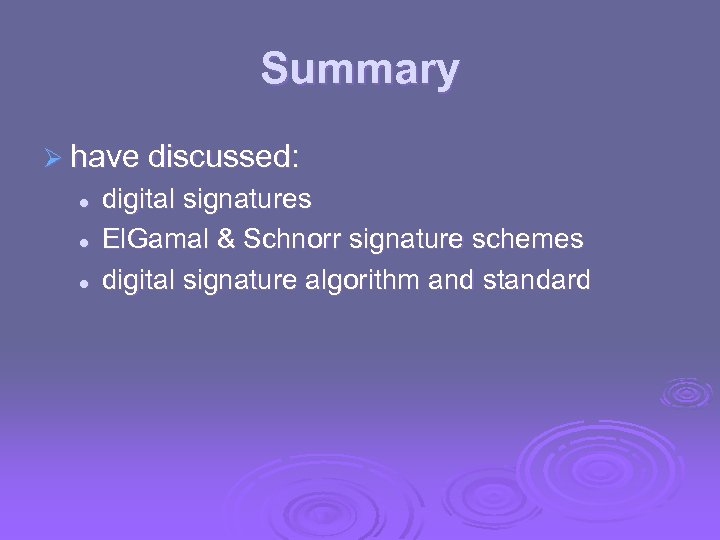Summary Ø have discussed: l l l digital signatures El. Gamal & Schnorr signature schemes digital signature algorithm and standard